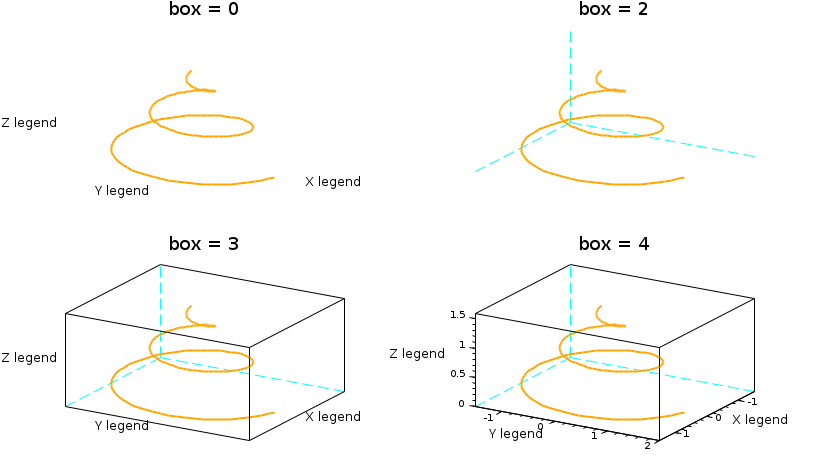Scilab Home page | Wiki | Bug tracker | Forge | Mailing list archives | ATOMS | File exchange
Change language to: English - Français - Português - Русский
Scilabヘルプ >> Graphics > 3d_plot > param3d

# param3d

plots a single curve in a 3D cartesian frame

# param3d1

plots a set of curves in a 3D cartesian frame. 1D mesh plot

### Syntax

```param3d(x, y, z)
param3d(x, y, z, [theta, alpha, leg, flag, ebox])
param3d // demo

param3d1(X, Y, Z)
param3d1(X, Y, list(Z, styles))
param3d1(.., [theta, alpha, leg, flag, ebox])
param3d1 // demo```

### Arguments

x, y, z

3 vectors of the same length: cartesian coordinates of the curve's points.

X, Y, Z

matrices of the same size (nl,nc). Each column #i of the matrices provides the coordinates of the points for the ith curve.

styles

vector of nc=size(Z,"c") integers specifying the style -- line color or mark style -- of each curve. When it is specified, it must be bundled with `Z` into a list, using `list(Z, styles)`.

By default, lines are drawn with colors indexed from 1 to nc in the current color map, without mark.

If `styles(i)<0`, the curve #i is plotted only with marks of style #|styles(i)|, without line.

Otherwise (`styles(i)>0`), the curve #i is rendered as a solid line of color #styles(i), without marks.

theta

Polar angle of the (OP) observation point to the north pole (Oz+), in degrees, from 0 to 180. Default = 35°.

alpha

Azimuth angle of the (OP) observation point, around the (OZ+) axis, in degrees. Default = 45°.

leg

single string setting the labels for the 3 axes. "@" is used as a labels separator. Default = "X@Y@Z"

flag = [type, box]

`type` and `box` have the same meaning as in `plot3d`:

type

Sets the axes scaling and bounding modes. Default = 2.

type gca().isoview data bounds
0 off [0,1, 0,1, -1,1]
1 off in `ebox`
2 off from data points
3 on in `ebox`
4 on from data points
5 on in `ebox`
6 on from data pointsIsometrical modes are rendered with `gca().cube_scaling="off"`.
box

an integer controlling the display of the axes frame (default = 4).

Value Displayed axes Axes legends gca().box gca().axes_visible
0 none. No frame. yes "off" "off"
1 not implemented. Like 0. Please use 0.
2 only axes lines on the back NO "hidden_axes" "off"
3 full ungraduated box yes "on" "off"
4 full box + 3 graduated axes yes "on" "on"

ebox

Vector `[xmin,xmax, ymin,ymax, zmin,zmax]` of axes bounds, used and assigned to `gca().data_bounds` when the `flag` option is used with the `type`=1|3|5. By default set to [0,1, 0,1, 0,1].

### Description

`param3d()` is used to plot a single 3D curve defined by its coordinates `x`, `y` and `z`.

The curve is an object of `polyline` type. When it is used just after `param3d()`, `gce`() returns the curve's identifier, from which all the curve's properties may be changed: color, line style, thickness, mark style and colors, etc.

`param3d1()` is used to plot a set of 3D curves, all having the same number of points. Then, `gce``().children` returns the vector of identifiers of the whole set of curves.The graphical identifier of the curve #i is `gce().children(\$-i+1)`.

### Examples

```t = 0:0.1:5*%pi;
r = (max(t)-t)/10;

clf
param3d(r.*sin(t), r.*cos(t), t/10, 30, 72,"X@Y@Z",[4,4])

curve = gce();
curve.mark_mode = "on";
curve.mark_style = 10;
curve.mark_foreground = color("magenta");```box parameter illustrated with param3d1() used for a single curve :

```t = (0:0.1:5*%pi)';
r = (max(t)-t)/10;
x = r.*sin(t); y = r.*cos(t); z = list(t/10, color("orange"));
Box = [0 2 3 4];

clf
for i = 1:4
subplot(2,2,i)
param3d1(x, y, z, 31, 72,"X legend@Y legend@Z legend",[4, Box(i)])
title(msprintf("box = %d",Box(i)), "fontsize", 4)
end
gcf().children.children.thickness = 2; // for the 4 curves in a once
gca().sub_ticks = [4 4 4];             // only for the last axes```param3d1() used for an actual set of curves:

```[X, Y] = ndgrid(-11:0.5:9);
R = sqrt(X.*X + Y.*Y) + %eps;
Z = sin(R)./R;

clf
subplot(1,3,1)
param3d1(X, Y, Z, 150, 85, flag=[2,4])

subplot(1,3,2)
param3d1(X, Y, Z, 150, 85, flag=[2,4])
gce().children.foreground = color("green");

subplot(1,3,3)
param3d1(X, Y, Z, 150, 85, flag=[2,4])
curves = gce().children;
curves(1:2:\$).foreground = color("orange");
gca().box = "back_half";

gcf().children.foreground = color("grey70"); // box & ticks color for the 3 figures``````[X, Y] = ndgrid(-11:0.5:9, -7:0.5:6); // x is the long side, y the short one
// => X has constant rows. Y has constant columns

clf

// Meshing lines at constant Y values
subplot(1, 3, 1)
R = sqrt(X.*X + Y.*Y) + %eps;
Z = sin(R)./R;
param3d1(X, Y, Z, 150, 85, flag=[2,4])

// Meshing lines at constant X values, with transposed X and Y:
subplot(1, 3, 2)
R = sqrt(X'.*X' + Y'.*Y') + %eps;
Z = sin(R)./R;
param3d1(X', Y', Z, 150, 85, flag=[2,4])

// With a curtain and filled curves to avoid messed overlaying parts
subplot(1, 3, 3)
[Xo, Yo] = ndgrid(-10:0.5:10);
R = sqrt(Xo.*Xo + Yo.*Yo) + %eps;
Zo = sin(R)./R;
// we add the curtain on starting and ending sides
nc = size(Xo,"c");
zmin = min(Zo);
X = [Xo(1,:) ; Xo ; Xo(\$,:)];
Y = [Yo(1,:) ; Yo ; Yo(\$,:)];
Z = [zmin*ones(1,nc) ; Zo ; zmin*ones(1,nc)];
// Rendering
param3d1(X, Y, Z, 150, 85, flag=[2,4])
e = gce();
e.children.fill_mode = "on";

// grey box for all axes:
gcf().children.foreground = color("grey70");```